### Example 40.10 Bayesian Analysis of a Poisson Regression Model

This example illustrates a Bayesian analysis of a log-linear Poisson regression model. Consider the following data on patients from clinical trials. The data set is a subset of the data described in Ibrahim, Chen, and Lipsitz (1999).

data Liver;
input X1-X6 Y;
datalines;
19.1358    50.0110     51.000      0        0       1        3
23.5970    18.4959      3.429      0        0       1        9
20.0474    56.7699      3.429      1        1       0        6
28.0277    59.7836      4.000      0        0       1        6
28.6851    74.1589      5.714      1        0       1        1
18.8092    31.0630      2.286      0        1       1       61
28.7201    52.9178     37.286      1        0       1        6
21.3669    61.6603     54.143      0        1       1        6
23.7332    42.2904      0.571      1        0       1       21
20.4783    22.1260     19.000      1        0       1        6

... more lines ...

17.0993    48.8384      3.000      0        0       0       9
19.1327    65.3425      2.571      1        0       0       1
17.3010    51.4493      4.429      1        0       0       6
;


The primary interest is in prediction of the number of cancerous liver nodes when a patient enters the trials, by using six other baseline characteristics. The number of nodes is modeled by a Poisson regression model with the six baseline characteristics as covariates. The response and regression variables are as follows:

 Y Number of Cancerous Liver Nodes X1 Body Mass Index X2 Age, in Years X3 Time Since Diagnosis of Disease, in Weeks X4 Two Biochemical Markers (each classified as normal=1 or abnormal=0) X5 Anti Hepatitis B Antigen X6 Associated Jaundice (yes=1, no=0)

Two analyses are performed using PROC GENMOD. The first analysis uses noninformative normal prior distributions, and the second analysis uses an informative normal prior for one of the regression parameters.

In the following BAYES statement, COEFFPRIOR=NORMAL specifies a noninformative independent normal prior distribution with zero mean and variance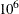for each parameter.

The initial analysis is performed using PROC GENMOD to obtain Bayesian estimates of the regression coefficients by using the following SAS statements:

proc genmod data=Liver;
model Y = X1-X6 / dist=Poisson link=log;
bayes seed=1 coeffprior=normal;
run;


Maximum likelihood estimates of the model parameters are computed by default. These are shown in the Analysis of Maximum Likelihood Parameter Estimates table in Output 40.10.1.

Output 40.10.1: Maximum Likelihood Parameter Estimates

The GENMOD Procedure

Bayesian Analysis

Analysis Of Maximum Likelihood Parameter Estimates
Parameter DF Estimate Standard Error Wald 95% Confidence Limits
Intercept 1 2.4508 0.2284 2.0032 2.8984
X1 1 -0.0044 0.0080 -0.0201 0.0114
X2 1 -0.0135 0.0024 -0.0181 -0.0088
X3 1 -0.0029 0.0022 -0.0072 0.0014
X4 1 -0.2715 0.0795 -0.4272 -0.1157
X5 1 0.3215 0.0832 0.1585 0.4845
X6 1 0.2077 0.0827 0.0456 0.3698
Scale 0 1.0000 0.0000 1.0000 1.0000

 Note: The scale parameter was held fixed.

Noninformative independent normal prior distributions with zero means and variances ofwere used in the initial analysis. These are shown in Output 40.10.2.

Output 40.10.2: Regression Coefficient Priors

The GENMOD Procedure

Bayesian Analysis

Independent Normal Prior for Regression
Coefficients
Parameter Mean Precision
Intercept 0 1E-6
X1 0 1E-6
X2 0 1E-6
X3 0 1E-6
X4 0 1E-6
X5 0 1E-6
X6 0 1E-6

Initial values for the Markov chain are listed in the Initial Values and Seeds table in Output 40.10.3. The random number seed is also listed so that you can reproduce the analysis. Since no seed was specified, the seed shown was derived from the time of day.

Output 40.10.3: MCMC Initial Values and Seeds

Initial Values of the Chain
Chain Seed Intercept X1 X2 X3 X4 X5 X6
1 1 2.450813 -0.00435 -0.01347 -0.00291 -0.27149 0.321507 0.207713

Summary statistics for the posterior sample are displayed in the Fit Statistics, Descriptive Statistics for the Posterior Sample, Interval Statistics for the Posterior Sample, and Posterior Correlation Matrix tables in Output 40.10.4, Output 40.10.5, Output 40.10.6, and Output 40.10.7, respectively. Since noninformative prior distributions for the regression coefficients were used, the mean and standard deviations of the posterior distributions for the model parameters are close to the maximum likelihood estimates and standard errors.

Output 40.10.4: Fit Statistics

Fit Statistics
DIC (smaller is better) 829.810
pD (effective number of parameters) 7.005

Output 40.10.5: Descriptive Statistics

The GENMOD Procedure

Bayesian Analysis

Posterior Summaries
Parameter N Mean Standard
Deviation
Percentiles
25% 50% 75%
Intercept 10000 2.4483 0.2320 2.2903 2.4493 2.6093
X1 10000 -0.00475 0.00809 -0.0101 -0.00466 0.000851
X2 10000 -0.0134 0.00237 -0.0150 -0.0134 -0.0118
X3 10000 -0.00303 0.00220 -0.00445 -0.00298 -0.00150
X4 10000 -0.2703 0.0799 -0.3241 -0.2725 -0.2190
X5 10000 0.3202 0.0828 0.2642 0.3209 0.3775
X6 10000 0.2106 0.0838 0.1533 0.2111 0.2663

Output 40.10.6: Interval Statistics

Posterior Intervals
Parameter Alpha Equal-Tail Interval HPD Interval
Intercept 0.050 1.9903 2.9059 2.0289 2.9321
X1 0.050 -0.0209 0.0108 -0.0211 0.0106
X2 0.050 -0.0181 -0.00870 -0.0184 -0.00908
X3 0.050 -0.00761 0.00105 -0.00745 0.00113
X4 0.050 -0.4257 -0.1063 -0.4314 -0.1152
X5 0.050 0.1563 0.4804 0.1574 0.4811
X6 0.050 0.0450 0.3777 0.0468 0.3788

Output 40.10.7: Posterior Sample Correlation Matrix

Posterior Correlation Matrix
Parameter Intercept X1 X2 X3 X4 X5 X6
Intercept 1.000 -0.708 -0.432 -0.046 -0.261 -0.185 -0.422
X1 -0.708 1.000 -0.202 -0.047 -0.035 0.078 0.129
X2 -0.432 -0.202 1.000 0.035 0.076 0.054 0.117
X3 -0.046 -0.047 0.035 1.000 0.027 -0.042 -0.077
X4 -0.261 -0.035 0.076 0.027 1.000 -0.024 0.127
X5 -0.185 0.078 0.054 -0.042 -0.024 1.000 -0.037
X6 -0.422 0.129 0.117 -0.077 0.127 -0.037 1.000

Posterior sample autocorrelations for each model parameter are shown in Output 40.10.8. The autocorrelation after 10 lags is negligible for all parameters, indicating good mixing in the Markov chain.

Output 40.10.8: Posterior Sample Autocorrelations

The GENMOD Procedure

Bayesian Analysis

Posterior Autocorrelations
Parameter Lag 1 Lag 5 Lag 10 Lag 50
Intercept 0.3037 0.0152 0.0095 -0.0170
X1 0.3398 0.0025 0.0003 0.0052
X2 0.3036 0.0061 0.0003 -0.0062
X3 0.3489 0.0190 -0.0064 -0.0210
X4 0.2868 0.0213 0.0157 -0.0107
X5 0.2854 0.0108 -0.0288 -0.0012
X6 0.3078 0.0230 0.0073 0.0062

The p-values for the Geweke test statistics shown in Output 40.10.9 all indicate convergence of the MCMC. See the section Assessing Markov Chain Convergence for more information about convergence diagnostics and their interpretation.

Output 40.10.9: Geweke Diagnostic Statistics

Geweke Diagnostics
Parameter z Pr > |z|
Intercept -0.6533 0.5135
X1 0.3418 0.7325
X2 0.3609 0.7182
X3 -0.3345 0.7380
X4 0.2851 0.7755
X5 -0.5266 0.5985
X6 1.1285 0.2591

The effective sample sizes for each parameter are shown in Output 40.10.10.

Output 40.10.10: Effective Sample Sizes

Effective Sample Sizes
Parameter ESS Autocorrelation
Time
Efficiency
Intercept 4880.3 2.0491 0.4880
X1 4844.2 2.0643 0.4844
X2 5139.3 1.9458 0.5139
X3 4551.2 2.1972 0.4551
X4 4953.6 2.0187 0.4954
X5 5330.5 1.8760 0.5331
X6 4988.1 2.0048 0.4988

Trace, autocorrelation, and density plots for the seven model parameters are shown in Output 40.10.11 through Output 40.10.17. All indicate satisfactory convergence of the Markov chain.

Output 40.10.11: Diagnostic Plots for Intercept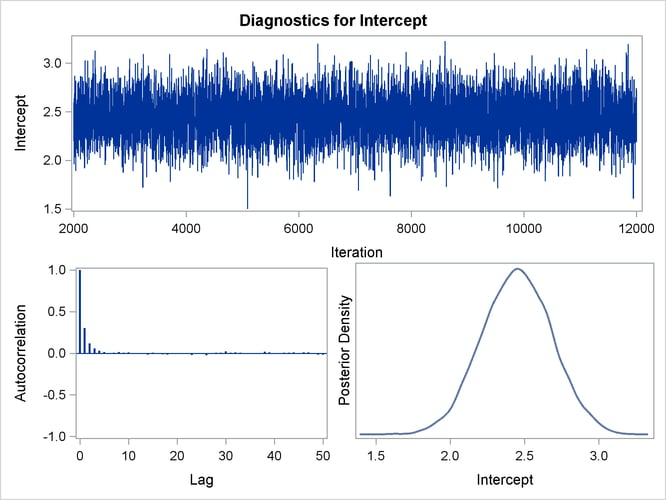Output 40.10.12: Diagnostic Plots for X1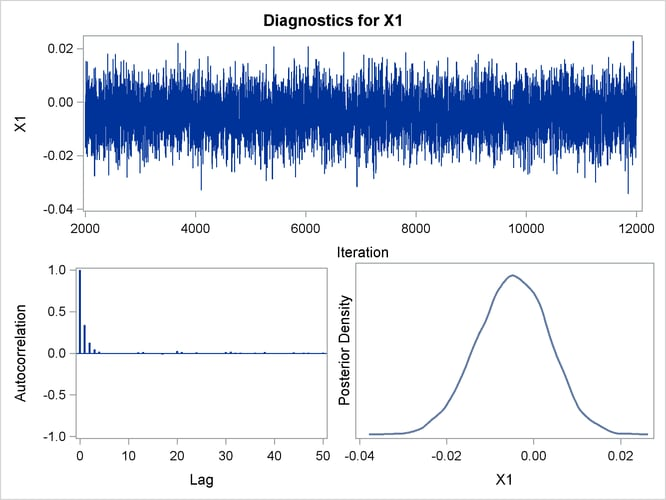Output 40.10.13: Diagnostic Plots for X2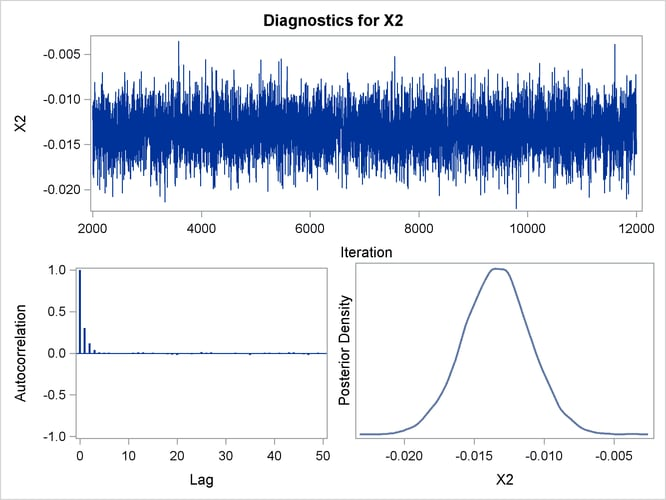Output 40.10.14: Diagnostic Plots for X3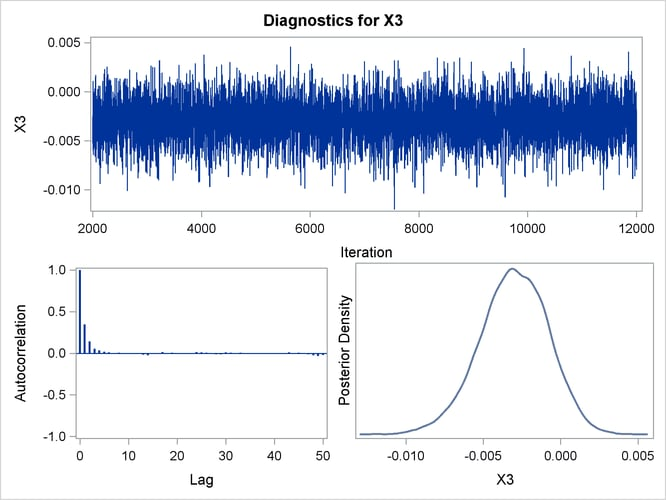Output 40.10.15: Diagnostic Plots for X4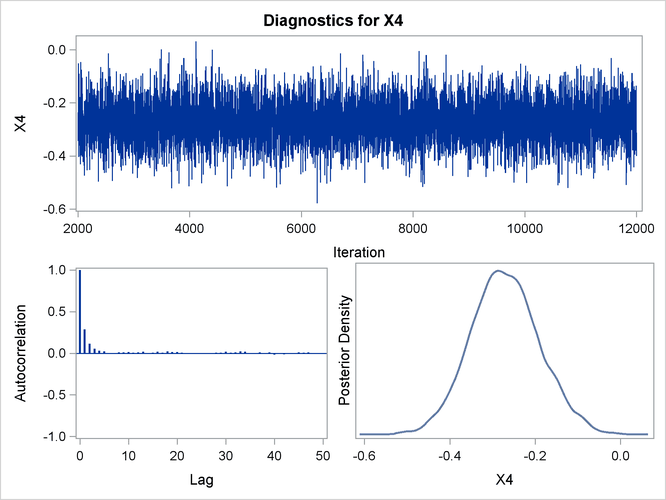Output 40.10.16: Diagnostic Plots for X5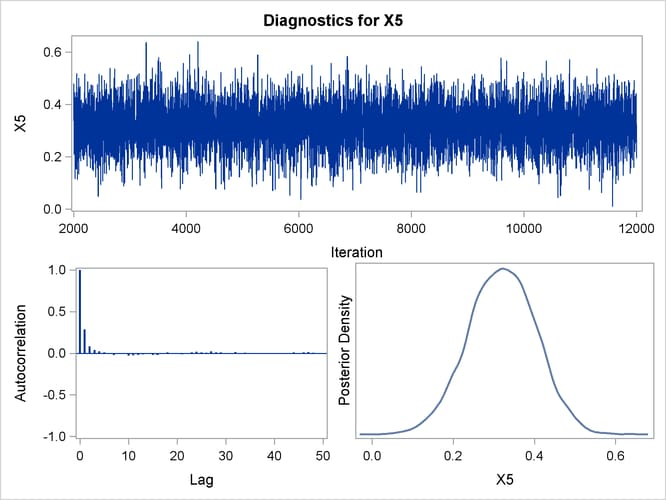Output 40.10.17: Diagnostic Plots for X6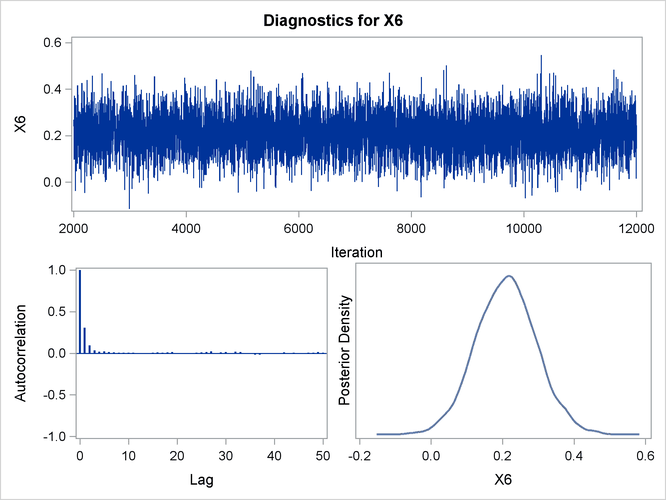In order to illustrate the use of an informative prior distribution, suppose that researchers expect that a unit increase in body mass index (X1) will be associated with an increase in the mean number of nodes of between 10% and 20%, and they want to incorporate this prior knowledge in the Bayesian analysis. For log-linear models, the mean and linear predictor are related by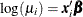. If X1and X1are two values of body mass index,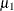and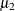are the two mean values, and all other covariates remain equal for the two values of X1, then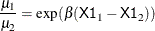so that for a unit change in X1,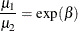If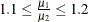, then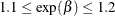, or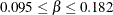. This gives you guidance in specifying a prior distribution for thefor body mass index. Taking the mean of the prior normal distribution to be the midrange of the values of, and taking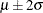to be the extremes of the range, an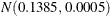is the resulting prior distribution. The second analysis uses this informative normal prior distribution for the coefficient of X1 and uses independent noninformative normal priors with zero means and variances equal tofor the remaining model regression parameters.

In the following BAYES statement, COEFFPRIOR=NORMAL(INPUT=NormalPrior) specifies the normal prior distribution for the regression coefficients with means and variances contained in the data set NormalPrior.

An analysis is performed using PROC GENMOD to obtain Bayesian estimates of the regression coefficients by using the following SAS statements:

data NormalPrior;
input _type_ \$ Intercept X1-X6;
datalines;
Var  1e6   0.0005      1e6      1e6      1e6      1e6      1e6
Mean 0.0   0.1385      0.0      0.0      0.0      0.0      0.0
;

proc genmod data=Liver;
model Y = X1-X6 / dist=Poisson link=log;
bayes seed=1 plots=none coeffprior=normal(input=NormalPrior);
run;


The prior distributions for the regression parameters are shown in Output 40.10.18.

Output 40.10.18: Regression Coefficient Priors

The GENMOD Procedure

Bayesian Analysis

Independent Normal Prior for Regression
Coefficients
Parameter Mean Precision
Intercept 0 1E-6
X1 0.1385 2000
X2 0 1E-6
X3 0 1E-6
X4 0 1E-6
X5 0 1E-6
X6 0 1E-6

Initial values for the MCMC are shown in Output 40.10.19. The initial values of the covariates are joint estimates of their posterior modes. The prior distribution for X1 is informative, so the initial value of X1 is further from the MLE than the rest of the covariates. Initial values for the rest of the covariates are close to their MLEs, since noninformative prior distributions were specified for them.

Output 40.10.19: MCMC Initial Values and Seeds

Initial Values of the Chain
Chain Seed Intercept X1 X2 X3 X4 X5 X6
1 1 2.14282 0.010595 -0.01434 -0.00301 -0.28062 0.334983 0.231213

Goodness-of-fit, summary, and interval statistics are shown in Output 40.10.20. Except for X1, the statistics shown in Output 40.10.20 are very similar to the previous statistics for noninformative priors shown in Output 40.10.4 through Output 40.10.7. The point estimate for X1 is now positive. This is expected because the prior distribution on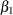is quite informative. The distribution reflects the belief that the coefficient is positive. Thedistribution places the majority of its probability density on positive values. As a result, the posterior density ofplaces more likelihood on positive values than in the noninformative case.

Output 40.10.20: Fit Statistics

Fit Statistics
DIC (smaller is better) 833.074
pD (effective number of parameters) 6.869

The GENMOD Procedure

Bayesian Analysis

Posterior Summaries
Parameter N Mean Standard
Deviation
Percentiles
25% 50% 75%
Intercept 10000 2.1419 0.2157 1.9965 2.1430 2.2894
X1 10000 0.0103 0.00684 0.00573 0.0104 0.0150
X2 10000 -0.0143 0.00233 -0.0159 -0.0142 -0.0127
X3 10000 -0.00318 0.00218 -0.00467 -0.00314 -0.00170
X4 10000 -0.2806 0.0800 -0.3336 -0.2793 -0.2266
X5 10000 0.3341 0.0832 0.2788 0.3341 0.3906
X6 10000 0.2333 0.0826 0.1774 0.2325 0.2880

Posterior Intervals
Parameter Alpha Equal-Tail Interval HPD Interval
Intercept 0.050 1.7225 2.5574 1.7293 2.5632
X1 0.050 -0.00344 0.0235 -0.00345 0.0234
X2 0.050 -0.0188 -0.00970 -0.0189 -0.00980
X3 0.050 -0.00757 0.00108 -0.00733 0.00121
X4 0.050 -0.4365 -0.1200 -0.4391 -0.1256
X5 0.050 0.1657 0.4966 0.1682 0.4987
X6 0.050 0.0695 0.3959 0.0725 0.3981